# Test: Heat

## 30 Questions MCQ Test Physics For JEE | Test: Heat

Description
Attempt Test: Heat | 30 questions in 60 minutes | Mock test for NEET preparation | Free important questions MCQ to study Physics For JEE for NEET Exam | Download free PDF with solutions
QUESTION: 1

### The compounds C2H5OC2H5 and CH3OCH2CH2CH3 are

Solution:

Here, ether is functional group and whenever there is change around same functional group they are metamers to each other.
These are chain as well as metamers but priority is given to metamerism even if we have to choose between metamer and position then, again metamer is to be opted first.

QUESTION: 2

### The number of isomers of pentane is

Solution:

Pentane (C5H12) is an organic compound with five carbon atoms. Pentane has three structural isomers that are n-pentane, Iso-pentane (methyl butane) and neopentane (dimethylpropane).

QUESTION: 3

### How many minimum no. of C-atoms are required for position & geometrical isomerism in alkene?

Solution:

The smallest alkene showing geometrical isomerism is but−2−ene (4−C atoms)
The smallest alkene showing position isomerism is butene (4−C atoms), where the position of double bonds may vary.

QUESTION: 4

How many structural formula are possible when one of the hydrogen is replaced by a chlorine atom in anthracene?

Solution: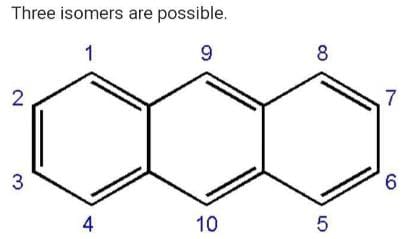Position 1,4,5,8 are equal.
Similarly 2,3,6,7 are equal.
Position 9 and 10 are equal.
So we will get three possible isomers

QUESTION: 5

Which of the following connot be written in an isomeric form?

Solution:

CH3​−CH(OH)−CH2​−CH3​ can have position isomer.
CH3​−CHO can have functional isomers (enol).
Cl−CH2​CH2​−Cl can have position isomer.
But CH2​=CH−Cl has one isomer but it is not preferred IUPAC name.

QUESTION: 6

The number of cis-trans isomer possible for the following compound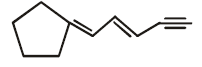Solution: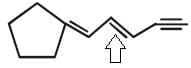Since only this is a double bond with different groups attached so only it will show cis-trans isomerism

QUESTION: 7

The number of isomers of dibromoderivative of an alkene (molear mass 186 g mol–1) is

Solution: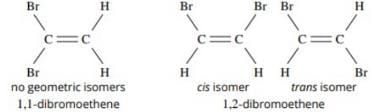QUESTION: 8

Increasing order of stability among the three main conformation (i.e. eclipse, anti, gauche) of ethylene glycol is:

Solution:

In the anti conformation the atoms are far apart ergo causing minimum repulsion whereas the opposite is true for eclipsed conformation. Gauche is in an in between stage.

QUESTION: 9

How many primary amines are possible for the formula C4H11N?

Solution:

The following 4 structures are possible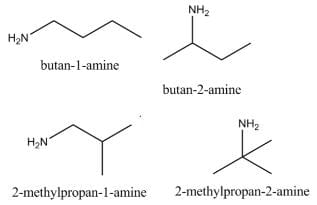QUESTION: 10

The R/S configuration of these compounds are respectively.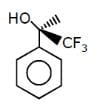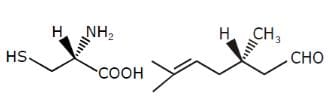Solution: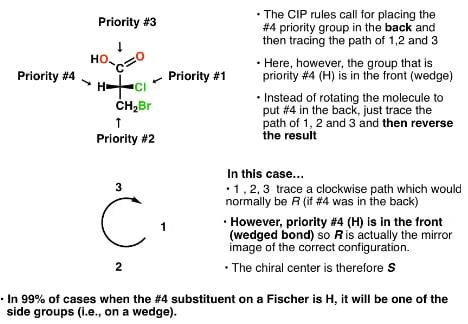Applying the previously stated results we get A as the answer

QUESTION: 11

How many planes (pos) are presents in Anthracene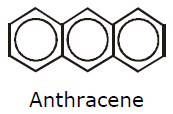Solution: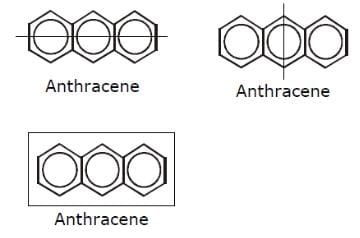QUESTION: 12

Two neighbours of homologous series differ by

Solution:

A homologous series is a series of compounds in which each member differs from the next/previous by CH2 or 14 mass units. Physical properties change in a homologous series but chemical properties remain almost the same because the functional group does not change.

QUESTION: 13

Geometrical isomers can be

Solution:

Diastereomers are isomers which are not mirror images of each other, while enantiomers are those which are mirror images of each other. A geometric isomer without any chiral centre is a mirror image of itself. Also, 2 geometric isomers, which are not mirror images of each other will be diastereomers.
Hence, geometrical isomers can be diastereomers or enantiomers.

QUESTION: 14

Phenol and benzyl alcohol are

Solution:

Phenol is C6​H5​(OH) while benzyl alcohol is C6​H5​(CH2​OH).
Here there is a difference of −HC2​ between their molecular formula.
According to the definition of homologous series, the adjacent members of the series differ by −CH2​ group.
Thus, Phenol and benzyl alcohol are homologous of each other.

QUESTION: 15

C3H8 belongs to the homologous series of

Solution:

C3H6 and C4H8 belongs to same homologous series as they both belongs to alkene series whose formula is CnH2n, here n = no. of carbon atoms.

QUESTION: 16

Which of the follownig compounds is (S)–4-chloro-1-methylcyclohexene ?

Solution:

Because when you see the question there is 1-methylcyclohexene this means the first numbering goes to methyl group and 'ene' group that is double bond and then it goes to 4 chloro that means from up to down including double bond we have to take chlorine on 4th position and it is (s) that means it is above the surface.

QUESTION: 17

Which of the following compounds has two stereogenic centers (asymmetric carbons) ?

Solution:

Asymmetric carbon is the carbon which has all the four attached groups different.And these carbons are also called chiral carbons.
Hence option A has asymmetric or chiral carbon.
Hence option A is correct.

QUESTION: 18

Which of the following structures represents a chiral compound ?

Solution:

This compound is chiral because its molecule has two chiral carbon atoms (C−3 and C-4).
ether molecules are chiral due to the presence of a plane of symmetry.

QUESTION: 19

Examine the compound on the right. How many stereoisomers having this constitution are possible ?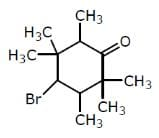Solution:

Explanation : 3 chiral centres

Number of Stereoisomers = 2n

= 23

= 8

QUESTION: 20

Which of the following heptanols are chiral 1-heptanol, 2-heptanol, 3-heptanol, 4-heptanol.

Solution:

As you can see, the third carbon atom from the left has three different groups attached to it namely 1. hydroxyl groups attached to namely 1. hydroxyl,
2. ethyl
3. n-butyl,
4. hydrogen
Hence the mentioned carbon atom is a chiral centre. Now any molecule having one chiral centre is a chiral molecule, i.e. 2 forms namely (R) and (S) forms of the molecule exist.
Please note that if the molecule has more than one chiral centre, We cannot directly conclude that the molecule will be chiral.

QUESTION: 21

Which of the following will exhibit geometrical isomerism ?

Solution:

1-phenyl-2-butene shows geometrical isomerism in the form of cis and trans isomers.
However, the geometrical isomerism is not possible for 3-phenyl-1-butene, 2-phenyl-1-butene and 1,1-diphenyl-1-propane.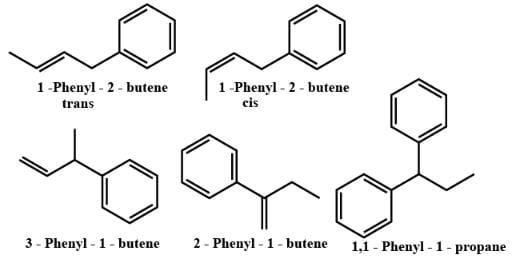QUESTION: 22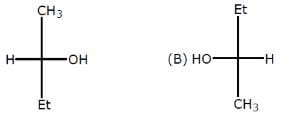Relation between given pair is

Solution:

The above pairs are known as identical pair. An isomer of a molecule has the number of atoms of each element but has a  different arrangement of the atoms.It has  the same molecular  formula as the other molecule , with a different chemical structure.

QUESTION: 23

Following eclipsed form of propane is repeated after rotation of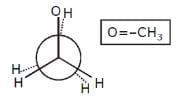Solution:
QUESTION: 24

Stereoisomers differ from each other in what respect ?

Solution:

Stereoisomers. Generally defined, stereoisomers are isomers that have the same composition (that is, the same parts) but that differ in the orientation of those parts in space.

QUESTION: 25

The number of isomers of C5H10 is

Solution:

Explanation : Given molecular formula is C5H10.

⇒ There are 6 isomeric alkenes by including Cis/trans isomers of pent−2−ene.

⇒ 7 Cycloalkanes also possible.

Totally it have 13 isomers.

QUESTION: 26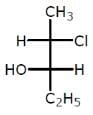The compound with the above configuration is called.

Solution:

4th priority group always lie in vertical
Clockwise: R
Anticlockwise: S
At 2nd carbon 1 → 2 → 3 configuration is r but 4 groups are horizontal so conf. change from R to s
At 3rd carbon a → b → c conf. is r but d group is horizontal so conf. change from R to S
Hence,
2s,3s)-c-chloro-3 hydropentane

QUESTION: 27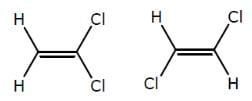The above compounds differ in

Solution:

The first compound is in cis phase and the other one is trans phase. they differ in their structure.

QUESTION: 28

The compound C2H5OC2H5 and CH3OCH2CH2CH3 are

Solution:

The compounds C2​H5​OC2​H5​ and CH3​OCH2​CH2​CH3​ are metamers.
They differ in the nature of the alkyl groups.

QUESTION: 29

Which conformer of cyclohexane is chiral

Solution:

We know that if a compound is symmetrical then then it is not chiral. hence chair conformation and boat conformations. Both are achiral whereas twist boat is chiral.

QUESTION: 30

Minimum C atoms required for a compound to show geometrical isomerism :

Solution: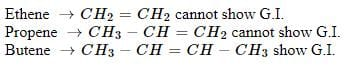Use Code STAYHOME200 and get INR 200 additional OFF Use Coupon Code

### How to Prepare for NEET

Read our guide to prepare for NEET which is created by Toppers & the best Teachers